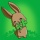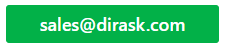Home
IT Knowledge
IT Career
Inspiration
Languages
EN

# Java random number between 1 and 10 - both inclusive

6 points
Created by:JustMike
26150

Hi, today we would like to show you how to generate random number in range between 1 and 10.

Truth be told the below code can be modified and used to generate random number between any range.

``````import java.util.Random;

public class RandomUtil {

private static final Random RANDOM = new Random();

public static int nextIntBetweenMinInclusiveMaxInclusive(int min, int max) {
return RANDOM.nextInt((max - min) + 1) + min;
}

public static void main(String[] args) {

for (int i = 0; i < 15; i++) {
int randomNumber = nextIntBetweenMinInclusiveMaxInclusive(1, 10);

System.out.println(randomNumber);
}
}
}``````

Output:

``````9
3
7
10
8
3
6
10
8
8
5
3
10
1
10``````

NOTE:

Each time click run this code we will see different results (statistically).

If we want to generate random int between different range we need to change min and max arguments we pass to the method:

Generate random int between 1 and 10:

``nextIntBetweenMinInclusiveMaxInclusive(1, 10);``

Generate random int between 5 and 30:

``nextIntBetweenMinInclusiveMaxInclusive(5, 30);``

And so on.

1. Java - random int number in both inclusive range with threads